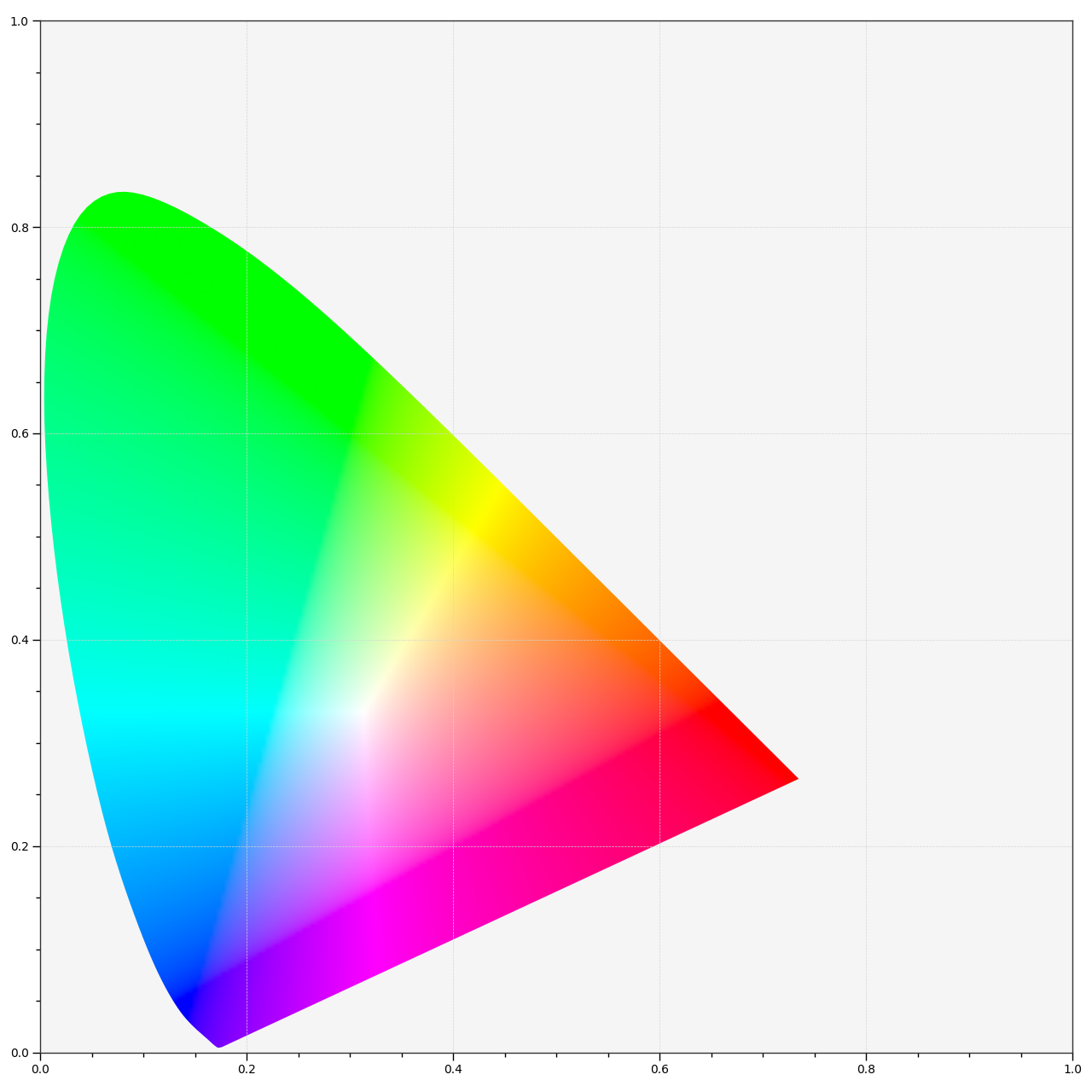# colour.plotting.diagrams.plot_chromaticity_diagram_colours#

colour.plotting.diagrams.plot_chromaticity_diagram_colours(samples: int = 256, diagram_colours: ArrayLike | str | None = None, diagram_opacity: float = 1, diagram_clipping_path: ArrayLike | None = None, cmfs: = 'CIE 1931 2 Degree Standard Observer', method: Literal['CIE 1931', 'CIE 1960 UCS', 'CIE 1976 UCS'] | str = 'CIE 1931', **kwargs: Any) Tuple[plt.Figure, plt.Axes][source]#

Plot the Chromaticity Diagram colours according to given method.

Parameters:
• samples (int) – Samples count on one axis when computing the Chromaticity Diagram colours.

• diagram_colours (ArrayLike | str | None) – Colours of the Chromaticity Diagram, if `diagram_colours` is set to RGB, the colours will be computed according to the corresponding coordinates.

• diagram_opacity (float) – Opacity of the Chromaticity Diagram.

• diagram_clipping_path (ArrayLike | None) – Path of points used to clip the Chromaticity Diagram colours.

• cmfs (MultiSpectralDistributions | str | Sequence[MultiSpectralDistributions | str]) – Standard observer colour matching functions used for computing the spectral locus boundaries. `cmfs` can be of any type or form supported by the `colour.plotting.common.filter_cmfs()` definition.

• method (Literal['CIE 1931', 'CIE 1960 UCS', 'CIE 1976 UCS'] | str) – Chromaticity Diagram method.

• kwargs (Any) – {`colour.plotting.artist()`, `colour.plotting.render()`}, See the documentation of the previously listed definitions.

Returns:

Current figure and axes.

Return type:

`tuple`

Examples

```>>> plot_chromaticity_diagram_colours(diagram_colours="RGB")
...
(<Figure size ... with 1 Axes>, <...Axes...>)
```